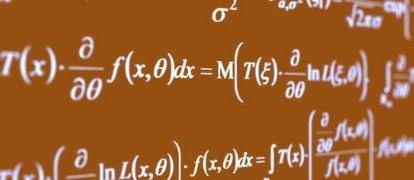# 6 Most Common Maths Ext 2 Integral Forms

In this post, we run through the most common Maths Extension 2 Integral forms so you can demonstrate that you are an exceptional student, and not just a knowledgeable one.The integration methods taught in Extension 2 can appear easy enough to grasp, but when it comes to the HSC, integration problems are sometimes deviously disguised by examiners to sort out the knowledgeable students from the exceptional ones.  It is important to know in detail the different forms of integrals and the methods used to approach them, so that when you are faced with an alien-looking expression, you are able to identify a suitable method to solve it.

Below are six of the most common forms of integrals, and examples of ways in which they can be disguised.

Can you solve all 6?

## 1. Reverse Chain Rule

When one part of the integer and is the derivative of the other part, the solution to the integral may be found by an application of the reverse chain rule.  Note that in special cases, this may also lead to a logarithm.  The reverse chain rule can be summarised as:

$$\int nf(x){^{n-1}}f {^\prime} (x) dx= f (x){^n}+C$$

### Example 1:

$$\int\frac{1}{xlnx}dx$$

### Example 2:

$$\int \frac{\sin x -\cos x}{(\sin x + cosx){^2}}dx$$

## 2. Standard Integrals

Before getting too stuck into a complicated-looking expression, don’t forget to check the table of standard integrals!  Usually, questions that can be solved using the table of standard integrals are worth fewer marks.

### Example 3:

$$\int \frac{dx}{9\cos{^2}x+\sin{^2}x}$$

## 3. Integration by Parts

$$\int u\frac{dv}{dx}=uv- \int v \frac{du}{dx}dx$$

A key indicator of an integration by parts problem is that the integral consists of one class of function (e.g. exponentials) multiplied by another (e.g. polynomials).  A classic example of this is

$$\int x \ e{^x} \ dx$$

However, it is important to note that just because there are two different classes of functions multiplied together, it doesn’t necessarily mean that it is an integration by parts problem; always check for the possibility of the reverse chain rule before attempting this method.

Sometimes, it is not so obvious where the two ‘parts’ of the integral are.  Consider the following example:

### Example 4:

$$\int \sec{^3}x \ dx$$

## 4. Partial Fractions

When the integral is expressed as one polynomial divided by another, first check for an application of the reverse chain rule or for a potential inverse tan integral.  If none of these work, then it is most likely a partial fractions problem.

In these cases, the integrand can be decomposed into partial fractions which are much simpler to integrate.  The procedure for finding a partial fractions decomposition involves rewriting each of the denominator parts as follows:

 $$\frac {1}{(x+1)(x+2)} = \frac{A}{x+1}+\frac{B}{x+2}$$
 $$\frac {1}{(x{^2}+1)(x+4)} = \frac{Ax + B}{x{^2}+1}+\frac{C}{x+4}$$
 $$\frac {1}{(x+1){^2}(x+2){^3}} = \frac{A}{x+1}+\frac{B}{(x+1){^2}}+\frac{C}{(x+2)}+\frac{D}{(x+2){^2}}+\frac{E}{(x+2){^3}}$$
 $$\frac {1}{(x+1){^2}(x+2){^2}} = \frac{Ax+B}{x{^2}+2}+\frac{Cx+D}{(x{^2}+2){^2}}+\frac{Ex+F}{(x{^2}+2){^3}}$$

### Example 5:

$$\int \frac{x+2}{x(x{^2}-1)}dx$$

## 5. The t-method

The t-formulas can be used to solve integrals in the form:

$$\int \frac{dx}{a \sin x + b \cos x + c}$$

Important relationships:

when $$t= \tan \frac{x}{2}$$

$$\sin x = \frac {2t}{1+t{^2}}$$

$$\cos x= \frac {1-t{^2}}{1+t{^2}}$$

$$\tan x = \frac {2t}{1-t{^2}}$$

Where $$dx= \frac{2dt}{1+t{^2}}$$

### Example 6:

$$\int \frac{dx}{3+2 \sin 2x}$$

## 6. Integrals Requiring Trigonometric Substitutions

Some types of integrals can be solved by using a substitution involving a trigonometric function. The usual indicator of a question requiring trigonometric substitution is the presence of a square root containing a sum or difference of perfect squares:

$$\sqrt {a{^2}\pm x{^2}}$$

Here are the common types of integrals, and the substitution that can be used to solve them:

$$\int x{^2} \sqrt{a{^2}-x{^2}} dx$$ or $$\int \frac{x{^2}}{\sqrt{a{^2}+x{^2}}} \rightarrow$$use $$x = a \sin \theta$$

$$\int x{^3} \sqrt{a{^2}+x{^2}} dx$$ or $$\int \frac{x{^3}}{\sqrt{a{^2}+x{^2}}} \rightarrow$$use $$x = a \tan \theta$$

Take a look at the two examples below.  Are they really very different?

### Example 7:

$$\int x{^3} \sqrt {1+x{^2}}dx$$

### Example 8:

$$\int e{^{4x}} \sqrt{1+e{^{2x}}}dx$$